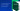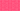Skip to contentPricingLoginSign up# Understanding Present Value and Net Present Value

As a company expands, significant decisions are made involving capital investment. Within financial analysis, present value and net present value are predominantly used to determine the feasibility and profitability of investment in a project or business. Both are important concepts to understand, and with the help of this simple guide, you’ll be able to calculate present value and net present value with skill and confidence.

## What is present value?

Present value, often abbreviated to PV, is the sum of an expected income stream or cash flow discounted at the present rate of return. Commonly used within investment analysis, financial planning, and risk management, it aids investors in determining whether or not the price they pay for an investment is appropriate or fair.

Knowing how to calculate present value will allow you to also calculate present value of annuity and present value of perpetuity. What’s more, it will help you to understand net present value, which we will look at later, as well as other key concepts such as discounted cash flow and internal rate of return.

## How to calculate present value

As financial formulas go, present value is a relatively simple one to understand. To calculate it, you need the estimated future value (FV), required rate of return (r) and the number of periods (n).

Present value = FV / (1 + r)n

Here’s an example:

If you expect to receive a cash flow of £100,000 after 5 years, at a 12% annual return, what would the present value of the investment be? Let’s follow the formula.

Present value = \$100,000 / (1 + 12%)5

The calculation gives us \$56,742.69, which means the current value of the investment today is \$56,742.69. When you look at it in reverse, if you invested this amount at 12% over 5 years, you’d have \$100,000.

You can work out present value on a calculator, if you have one to hand. Alternatively, you can try one of the many online present value calculators available.

## What is net present value?

If you need to compare projects and decide which ones to pursue, there are generally three routes to go down: determining the internal return, payback method, or net present value. Net present value, also referred to as NPV, is the present value of the cash flows at the required rate of return of the project compared to the initial investment. As its name suggests, net present value is the net of the present value of cash inflows and outflows by discounting all of the flows at a specified rate.

When looking at the numbers, a positive NPV is good, indicating that an investment is earning more than the discount rate. A negative NPV, on the other hand, is bad as it indicates that an investment is earning less than the discount rate, despite earning a positive rate.

## How to calculate net present value

To calculate the net present value, you need the estimated periodic net cash flow (R), the required rate of return (i), and the number of time periods (n). The net present value formula is:

Net present value = R x 1 - (1 + i)-n / I - initial investment

Here’s an example:

How would you calculate the net present value of a project which requires an initial investment of £243,000 and is expected to generate a net cash flow of £50,000 each month for 12 months? Let’s say that the target rate of return is 12% per annum.

= \$50,000 × (1 − (1 + 1%)-12) ÷ 1% − \$243,000

= \$50,000 × (1 − (1 + 1%)^-12) ÷ 1% − \$243,000

= \$50,000 × (1 − 1.01^-12) ÷ 0.01 − \$243,000

≈ \$50,000 × (1 − 0.887449) ÷ 0.01 − \$243,000

≈ \$50,000 × 0.112551 ÷ 0.01 − \$243,000

≈ \$50,000 × 11.2551 − \$243,000

≈ \$562,754 − \$243,000

≈ \$319,754

You can also use an online net present value calculator to quickly find out the NPV, but always ensure it comes from a trusted source.

## We can help

GoCardless helps you automate payment collection, cutting down on the amount of admin your team needs to deal with when chasing invoices. Find out how GoCardless can help you with ad hoc payments or recurring payments.

GoCardless makes it easy to collect recurring payments

Sign upContact sales

Contact Us

Sales

Contact sales

Support

Seen 'GoCardless Ltd' on your bank statement? Learn more

GoCardless Ltd., 10-20 Gwynne St, Cremorne, VIC 3121, Australia

GoCardless (company registration number 07495895) is authorised by the Financial Conduct Authority under the Payment Services Regulations 2017, registration number 597190, for the provision of payment services.